Next: 2-d problem with Neumann Up: Poisson's equation Previous: An example solution of

## 2-d problem with Dirichlet boundary conditions

Let us consider the solution of Poisson's equation in two dimensions. Suppose that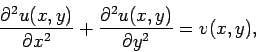(146)

for, and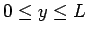. By direct analogy with our previous method of solution in the 1-d case, we could discretize the above 2-d problem using a second-order, central difference scheme in both the- and-directions. Unfortunately, such a discretization scheme yields a set of equations which cannot be reduced to a simple tridiagonal matrix equation. In fact, all of the efficient numerical algorithms for solving this type of problem are iterative in nature. For instance, the Jacobi method, the Gauss-Seidel method, the successive over-relaxation method, and the multi-grid method.34Regrettably, unless such iteration methods are extremely sophisticated (e.g., the multi-grid method), and, hence, beyond the scope of this course, they tend to converge very poorly. In the following, rather than discuss iterative methods which do not work very well, we shall instead discuss a non-iterative method which works effectively for a restricted set of problems. The method in question is termed a spectral method, since it involves expanding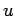andas truncated Fourier series in the-direction.

Suppose that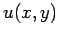satisfies mixed boundary conditions in the-direction: i.e.,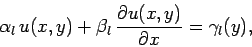(147)

at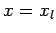, and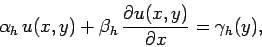(148)

at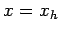. Here,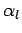,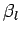, etc., are known constants, whereas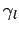,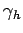are known functions of. Furthermore, suppose thatsatisfies the following simple Dirichlet boundary conditions in the-direction: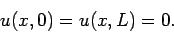(149)

Note that, sinceis a potential, and, hence, probably undetermined to an arbitrary additive constant, the above boundary conditions are equivalent to demanding thattake the same constant value on both the upper and lower boundaries in the-direction.

Let us writeas a Fourier series in the-direction: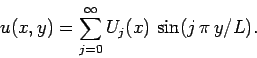(150)

Note that the above expression forautomatically satisfies the boundary conditions in the-direction. The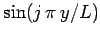functions are orthogonal, and form a complete set, in the interval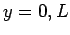. In fact,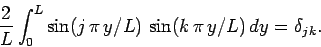(151)

Thus, we can write the source term as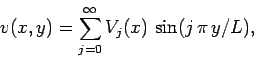(152)

where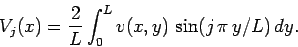(153)

Furthermore, the boundary conditions in the-direction become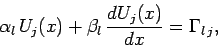(154)

at, and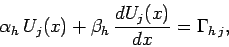(155)

at, where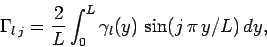(156)

etc.

Substituting Eqs. (150) and (152) into Eq. (146), and equating the coefficients of the(since these functions are orthogonal), we obtain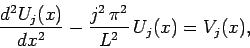(157)

for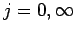. Now, we can discretize the problem in the-direction by truncating our Fourier expansion: i.e., by only solving the above equations for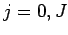, rather than. This is essentially equivalent to discretization in the-direction on the equally-spaced grid-points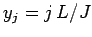. The problem is discretized in the-direction by dividing the domain into equal segments, according to Eq. (114), and approximating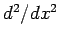via the second-order, central difference scheme specified in Eq. (115). Thus, we obtain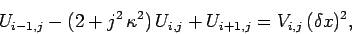(158)

for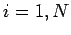and. Here,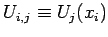,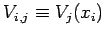, and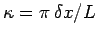. The boundary conditions (154) and (155) discretize to give: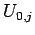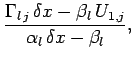(159)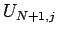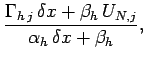(160)

for. Eqs. (158), (159), and (160) constitute a set of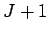uncoupled tridiagonal matrix equations (with one equation for each separate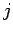value). These equations can be inverted, using the algorithm discussed in Sect. 5.4, to give the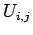. Finally, the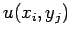values can be reconstructed from Eq. (150). Hence, we have solved the problem.Next: 2-d problem with Neumann Up: Poisson's equation Previous: An example solution of
Richard Fitzpatrick 2006-03-29